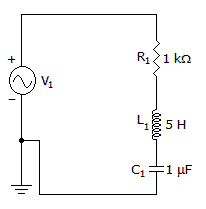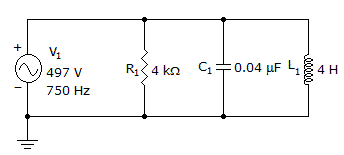# Electronics - RLC Circuits and Resonance

### Exercise :: RLC Circuits and Resonance - General Questions

6.

What is the range between f1 and f2 of an RLC circuit that resonates at 150 kHz and has a Q of 30?

 A. 100.0 kHz to 155.0 kHz B. 147.5 kHz to 152.5 kHz C. 4500 kHz to 295.5 kHz D. 149,970 Hz to 150,030 Hz

Answer: Option B

Explanation:

No answer description available for this question. Let us discuss.

7.

What is the quality factor?A. 999 B. 223 C. 2.2 D. 0.99

Answer: Option C

Explanation:

No answer description available for this question. Let us discuss.

8.

What effect will a parallel tank have upon final filter current?

 A. very little B. The bandpass frequencies will change. C. The frequency cutoff will change. D. The impedance will block output.

Answer: Option A

Explanation:

No answer description available for this question. Let us discuss.

9.

What is the total current?A. 56.6 mA B. 141 mA C. 191 mA D. 244 mA

Answer: Option B

Explanation:

No answer description available for this question. Let us discuss.

10.

When a parallel circuit resonates it is said to:

 A. flywheel B. oscillate C. both of the above D. none of the above

Answer: Option C

Explanation:

No answer description available for this question. Let us discuss.

#### Current Affairs 2021

Interview Questions and Answers